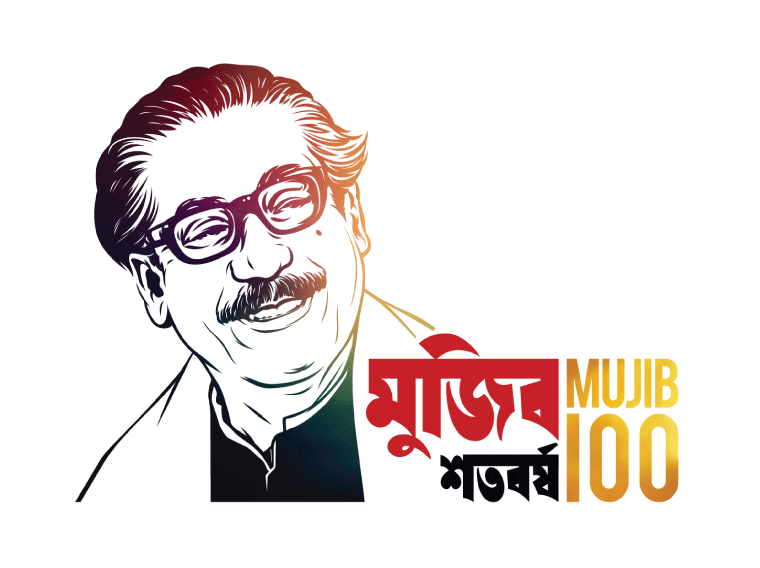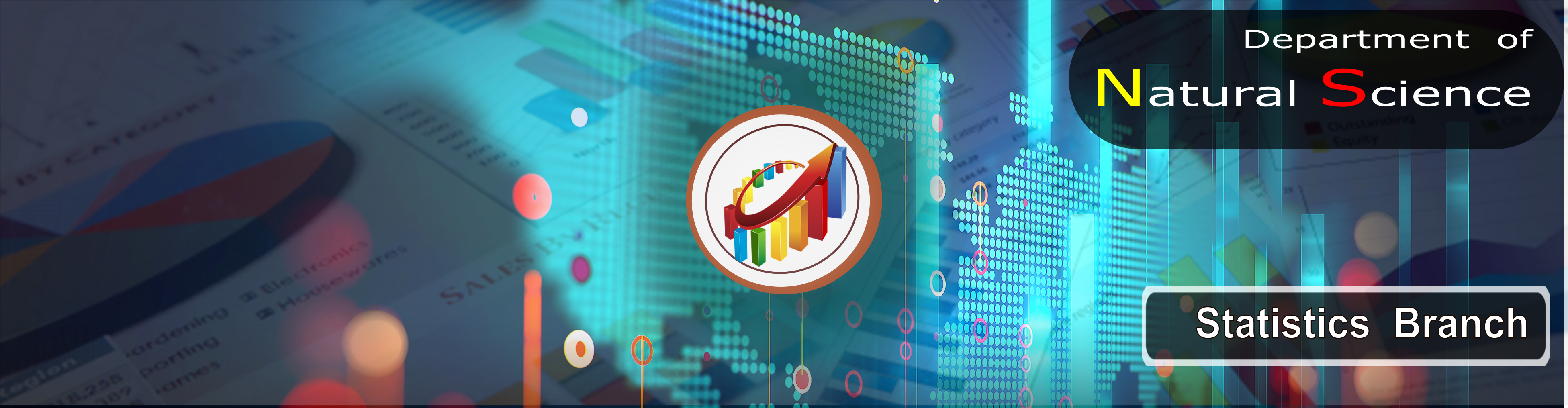Welcome to Port City International University#### Course of statistics

Statistics courses help the students to know about basic concepts of statistics and to apply in practical fields. After going through these subjects one can know about the quality of data, summarizing and analyzing the data. Knowledge of statistics is very helpful for forecasting.

Main Courses

4. Basic Math & Statistics   (MATH 121) ― Fashion Design & Technology
5. Introduction to Statistics (BPJ 209) ― Journalism and Media Studies
6. Statistics (STS 235) ― Computer Science & Engineering
7. VA & Statistics   (Math 235) ― Civil Engineering
8. Statistics (Math 211) ― Textile Engineering
9. Probability & Statistics  (MAT 225) ― Electrical & Electronic Engineering
10. VA & Statistics (Math 235) ―Civil Engineering
11. Statistics (Math 211) ― Textile Engineering
12. Probability & Statistics (MAT 225) ―  Electrical & Electronic Engineering

#### Teachers

View Teacher List of Course of statistics